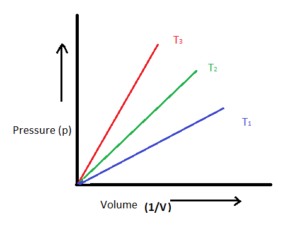### Gas laws

CHEMISTRY  SSS1 Second Term

WEEK 6

Gas laws

Performance Objectives

The student should be able to:

1. State Boyles and Charles law
2. Solve calculations on Boyle’s and Charles law
3. Solve calculations on general gas equation

Content

Boyle’s law

Boyle’s law states that the pressure of a fixed mass of gas is inversely proportional to the volume provided the temperature remains constant.Graphical representation of Boyle's law

I.e. P α 1/V

P = K/V

PV = K

Hence, P1V1 = P2V2

Worked example 1

200cm3 of a gas has a pressure of 500mmhg. What will.... View More